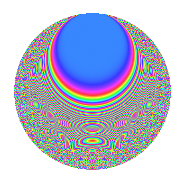# Properties

 Label 6017.2.a.eLevel 6017 Weight 2 Character orbit 6017.a Self dual Yes Analytic conductor 48.046 Analytic rank 0 Dimension 119 CM No

# Related objects

## Newspace parameters

 Level: $$N$$ = $$6017 = 11 \cdot 547$$ Weight: $$k$$ = $$2$$ Character orbit: $$[\chi]$$ = 6017.a (trivial)

## Newform invariants

 Self dual: Yes Analytic conductor: $$48.0459868962$$ Analytic rank: $$0$$ Dimension: $$119$$ Fricke sign: $$-1$$ Sato-Tate group: $\mathrm{SU}(2)$

## $q$-expansion

The dimension is sufficiently large that we do not compute an algebraic $$q$$-expansion, but we have computed the trace expansion.

 $$\operatorname{Tr}(f)(q) =$$ $$119q + 15q^{2} + 15q^{3} + 133q^{4} + 6q^{5} + 16q^{6} + 72q^{7} + 39q^{8} + 128q^{9} + O(q^{10})$$ $$\operatorname{Tr}(f)(q) =$$ $$119q + 15q^{2} + 15q^{3} + 133q^{4} + 6q^{5} + 16q^{6} + 72q^{7} + 39q^{8} + 128q^{9} + 22q^{10} + 119q^{11} + 40q^{12} + 67q^{13} + 3q^{14} + 22q^{15} + 145q^{16} + 57q^{17} + 53q^{18} + 68q^{19} + 25q^{20} + 21q^{21} + 15q^{22} + 21q^{23} + 34q^{24} + 137q^{25} + 10q^{26} + 54q^{27} + 149q^{28} + 46q^{29} + 10q^{30} + 87q^{31} + 58q^{32} + 15q^{33} + 16q^{34} + 40q^{35} + 137q^{36} + 39q^{37} + 27q^{38} + 72q^{39} + 46q^{40} + 50q^{41} - 4q^{42} + 122q^{43} + 133q^{44} + 12q^{45} + 22q^{46} + 92q^{47} + 9q^{48} + 161q^{49} + 2q^{50} - 12q^{51} + 177q^{52} + 12q^{53} + 19q^{54} + 6q^{55} - 16q^{56} + 43q^{57} + 56q^{58} + 39q^{59} + 27q^{60} + 114q^{61} + 66q^{62} + 196q^{63} + 161q^{64} + 7q^{65} + 16q^{66} + 59q^{67} + 139q^{68} - 24q^{69} + 9q^{70} + 11q^{71} + 92q^{72} + 123q^{73} + q^{74} + 19q^{75} + 92q^{76} + 72q^{77} - 101q^{78} + 78q^{79} - 34q^{80} + 139q^{81} + 73q^{82} + 108q^{83} - 31q^{84} + 30q^{85} - 18q^{86} + 164q^{87} + 39q^{88} + 15q^{89} - 41q^{90} + 60q^{91} - 26q^{92} - 2q^{93} + 45q^{94} + 75q^{95} + 42q^{96} + 73q^{97} + 32q^{98} + 128q^{99} + O(q^{100})$$

## Embeddings

For each embedding $$\iota_m$$ of the coefficient field, the values $$\iota_m(a_n)$$ are shown below.

For more information on an embedded modular form you can click on its label.

Label $$a_{2}$$ $$a_{3}$$ $$a_{4}$$ $$a_{5}$$ $$a_{6}$$ $$a_{7}$$ $$a_{8}$$ $$a_{9}$$ $$a_{10}$$
1.1 −2.72929 2.06420 5.44901 −2.31705 −5.63378 0.650725 −9.41333 1.26091 6.32390
1.2 −2.72367 −2.85757 5.41840 −2.70389 7.78308 4.38831 −9.31062 5.16569 7.36452
1.3 −2.68163 0.369101 5.19115 2.09046 −0.989794 1.10498 −8.55750 −2.86376 −5.60585
1.4 −2.67204 2.89488 5.13980 2.14144 −7.73524 4.84141 −8.38968 5.38033 −5.72201
1.5 −2.64305 −0.959171 4.98572 −4.09680 2.53514 −1.46535 −7.89140 −2.07999 10.8280
1.6 −2.64280 −2.08644 4.98439 2.06491 5.51405 1.23299 −7.88714 1.35325 −5.45715
1.7 −2.59415 −0.508113 4.72962 4.04973 1.31812 4.63801 −7.08105 −2.74182 −10.5056
1.8 −2.46359 −1.49738 4.06928 −0.810928 3.68894 4.87400 −5.09785 −0.757845 1.99779
1.9 −2.40399 −2.26995 3.77918 −1.51011 5.45695 −2.08665 −4.27714 2.15269 3.63028
1.10 −2.39877 0.712166 3.75408 0.976168 −1.70832 −3.65167 −4.20764 −2.49282 −2.34160
1.11 −2.37466 0.769590 3.63899 −2.34020 −1.82751 −2.65740 −3.89204 −2.40773 5.55717
1.12 −2.35458 −1.66572 3.54404 1.38814 3.92207 −2.86994 −3.63557 −0.225374 −3.26849
1.13 −2.35115 2.19609 3.52790 0.407257 −5.16332 −2.35563 −3.59233 1.82279 −0.957522
1.14 −2.33754 1.15439 3.46412 −4.35932 −2.69844 3.66625 −3.42244 −1.66738 10.1901
1.15 −2.30862 2.25355 3.32972 2.61387 −5.20258 1.15866 −3.06981 2.07847 −6.03443
1.16 −2.22427 3.08840 2.94737 −2.11023 −6.86944 3.85755 −2.10721 6.53822 4.69372
1.17 −2.14072 −2.03723 2.58269 3.12940 4.36115 −2.52931 −1.24739 1.15032 −6.69918
1.18 −1.98872 0.557360 1.95500 0.637327 −1.10843 2.66044 0.0894893 −2.68935 −1.26746
1.19 −1.97087 1.79688 1.88432 −3.99793 −3.54141 −0.876225 0.227993 0.228774 7.87939
1.20 −1.94884 3.22760 1.79797 1.61357 −6.29006 −0.583291 0.393727 7.41737 −3.14458
See next 80 embeddings (of 119 total)
 $$n$$: e.g. 2-40 or 990-1000 Embeddings: e.g. 1-3 or 1.119 Significant digits: Format: Complex embeddings Normalized embeddings Satake parameters Satake angles

## Inner twists

This newform does not have CM; other inner twists have not been computed.

## Atkin-Lehner signs

$$p$$ Sign
$$11$$ $$-1$$
$$547$$ $$1$$

## Hecke kernels

This newform can be constructed as the intersection of the kernels of the following linear operators acting on $$S_{2}^{\mathrm{new}}(\Gamma_0(6017))$$:

 $$T_{2}^{119} - \cdots$$ $$T_{3}^{119} - \cdots$$